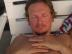## vec is slow, little-endian and limited

I remembered from the perl docs to use vec to compress arrays of integers to bitvectors. In my case with `Perfect::Hash::Urban` the size advantage is 3320 byte vs 88 bytes for two integer arrays of size 20 with values `[0..19]`, which compress to 8 bit per entry with vec. This is 300x less bytes. The theoretical maximal simple compression would be 13 byte, i.e. 5 bits per entry * 20 = 100 bits = 13 byte. Stored as Perl PV this would require 68 byte PV overhead + 13 byte for the bitvector.

But vec is only allowing sizes which are a power of 2, i.e. (1,2,4,8.16,32 and with 64 bit machines 64). So it seems to use natural words to store the bits, allowing fast access to each entry. With 64 bits it even throws a portable warning. I have no idea what this warning is for nowadays, other than Storable would not be able to thaw it on 32bit machines which do not support 64bit ints. Currently it hurts performance at every single get and set access at run-time for vec with 64 bits entries.

So I implemented my bitvector to store intermediate tables for my perfect hashes in pure-perl and XS with vec, and it was about 2x faster and 300x smaller. That's approximately what I expected.

``````# convert 2 int arrays @G and @V to one bitvector \$G (a string)
my \$bits = 1+length(sprintf "%b",\$size); # +1 for negative values
for (2,4,8,16,32,(\$Config{ptrsize}==8?(64):())) {
next if \$bits > \$_;
\$bits = \$_; last;
}
my \$G = "\0" x int(\$bits * \$size / 4); # calloc it
# \$G = @G
for my \$i (0..\$#G) {
vec(\$G, \$i, \$bits) = \$G[\$i] if \$G[\$i];
}
# \$G+offset = @V
for my \$i (0..\$#V) { # put V after G
vec(\$G, \$i+\$size, \$bits) = \$V[\$i] if \$V[\$i];
}
printf("\\$G\[\$bits]=\"%s\":%d\n", unpack("h*", \$G), length(\$G))
if \$options{-debug};
``````

and read-access is like this:

``````my \$v = vec(\$G, \$index, \$bits); # vec version of \$v = \$G[\$index];
``````

But then I tried perfect hashes on bigger dictionaries, like `/usr/share/dict/words` which need tables of 32 bits per entry, which are needed for 65535 to 4294967295 entries. Note that with that many entries a real bitvector, i.e. via Bit::Vector might be better than the simple approach with vec, which does not support bit sizes between 16 and 32 or even in 32 - 64. But so far I prefer to work without any external dependencies.

Everything works fine in pure-perl, but I wanted faster arithmetic and hash calculations in XS for bigger dictionaries. Like the CPAN Metadata hashes or the various perl5 unicode tables.

But my XS version did not work with bigger dictionaries, it only worked fine with 8 bit tables, which is for a maximum table size of 64.

My XS implementation of vec looked like this:

``````static inline
IV vec(char *G, IV index, IV bits) {
if (bits == 8)
return *(char*)(G + index) & 255;
else if (bits == 4)
return *(char*)(G + (index / 2)) & 15;
else if (bits == 16) {
short l = *(short*)((short*)G + index); /* __UINT16_MAX__ */
return (IV)l;
}
else if (bits == 32) {
#if INTSIZE == 4
int l = *(int*)((int*)G + index); /* __UINT32_MAX__ */
#else
long l = *(long*)((long*)G + index);
#endif
return (IV)l;
}
#ifdef HAVE_QUAD
else if (bits == 64) {
long l = *(long*)((long*)G + index);
return (IV)l;
}
#endif
}
``````

Every access translates to natural words and index offsets, which translates to a single assembler instruction per size. But I got overflows on most indices, so I looked up the perl5 implementation of vec in doop.c and started getting angry.

vec is really little-endian only, no natural endianness, so perl5 does byte fiddling for all the various sizes, but then the bit size limit makes no sense at all anymore, as the naive implementation supporting little-endian only would be a simple loop independent of the bits, instead of all the if branches to check the supported bit sizes. So perl5 vec is not only unnecessarily slow, and only supports little-endian, not native endian-ness, it also adds arbitrary limits on the supported bit sizes for no good technical reason.

So either use `Bit::Vector` or pack/unpack instead. vec is good for nothing. But I'll probably use just my fast nvec implementation for natural endian-ness and natural word sizes as shown above. This is a drop-in replacement for `vec` for bits>=4, but works with cooperative access for XS and Pure-Perl, and is fast.

## 5 Comments

When making my Data::BitStream and Data::BitStream::XS modules I did a bunch of vec() manipulation, including versions using Bit::Vector, strings, and my own XS.

In many cases what I found was that often just using string storage was faster than Bit::Vector, merely because Perl optimizes the heck out of things like substr. Once the vector grows large (e.g. for Unary codes) then Bit::Vector is better. Using 32-bit vectors with bit twiddling in Perl was pretty close to Bit::Vector's speed for my operations. Of course it will differ based on your operations.

Using an XS back end for the bit manipulation results in ~70x speedup for this application (and another 2x speedup if I go straight to XS and skip the Perl Moo class entirely, but then you give up some extensibility).

I've never actually found a use-case for vec() to do anything other than maintaining the bitvectors for a select() system call.

Nice finding! But since you have already done all this work, why not fixing vec in core instead of making a module?

### About Reini UrbanWorking at cPanel on cperl, B::C (the perl-compiler), parrot, B::Generate, cygwin perl and more guts, keeping the system alive.